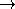### Definition

The class Min_quadrilateral_default_traits_2<K> is a traits class for the functions min_rectangle_2, min_parallelogram_2 and min_strip_2 using a two-dimensional CGAL kernel.

### Requirements

The template parameter K is a model for Kernel.

### Predicates

 Min_quadrilateral_default_traits_2::Equal_2 Kernel::Equal_2. Min_quadrilateral_default_traits_2::Less_xy_2 Kernel::Less_xy_2. Min_quadrilateral_default_traits_2::Less_yx_2 Kernel::Less_yx_2. Min_quadrilateral_default_traits_2::Orientation_2 Kernel::Orientation_2. Min_quadrilateral_default_traits_2::Has_on_negative_side_2 Kernel::Has_on_negative_side_2. Min_quadrilateral_default_traits_2::Compare_angle_with_x_axis_2 Kernel::Compare_angle_with_x_axis_2. Min_quadrilateral_default_traits_2::Area_less_rectangle_2 AdaptableBinaryFunction class op: Rectangle_2  × Rectangle_2bool. op(r1,r2) returns true, iff the area of r1 is strictly less than the area of r2. Min_quadrilateral_default_traits_2::Area_less_parallelogram_2 AdaptableBinaryFunction class op: Parallelogram_2  × Parallelogram_2bool. op(p1,p2) returns true, iff the area of p1 is strictly less than the area of p2. Min_quadrilateral_default_traits_2::Width_less_strip_2 AdaptableBinaryFunction class op: Strip_2  × Strip_2bool. op(s1,s2) returns true, iff the width of s1 is strictly less than the width of s2.

### Constructions

 Min_quadrilateral_default_traits_2::Construct_vector_2 Kernel::Construct_vector_2. Min_quadrilateral_default_traits_2::Construct_vector_from_direction_2 AdaptableFunctor op: Direction_2Vector_2. op(d) returns a vector in direction d. Min_quadrilateral_default_traits_2::Construct_perpendicular_vector_2 Kernel::Construct_perpendicular_vector_2. Min_quadrilateral_default_traits_2::Construct_direction_2 Kernel::Construct_direction_2. Min_quadrilateral_default_traits_2::Construct_opposite_direction_2 Kernel::Construct_opposite_direction_2. Min_quadrilateral_default_traits_2::Construct_line_2 Kernel::Construct_line_2. Min_quadrilateral_default_traits_2::Construct_rectangle_2 Function class op: Point_2  × Direction_2  × Point_2  × Point_2  × Point_2Rectangle_2. If the points p1,p2,p3,p4 form the boundary of a convex polygon (oriented counterclockwise), op(p1,d,p2,p3,p4) returns the rectangle with one of the points on each side and one sides parallel to d. Min_quadrilateral_default_traits_2::Construct_parallelogram_2 Function class op: Point_2  × Direction_2  × Point_2  × Direction_2  × Point_2  × Point_2Rectangle_2. If the points p1,p2,p3,p4 form the boundary of a convex polygon (oriented counterclockwise), op(p1,d1,p2,d2,p3,p4) returns the parallelogram with one of the points on each side and one side parallel to each of d1 and d2. Min_quadrilateral_default_traits_2::Construct_strip_2 Function class op: Point_2  × Direction_2  × Point_2Strip_2. op(p1,d,p2) returns the strip bounded by the lines through p1 resp. p2 with direction d.

### Operations

 template < class OutputIterator > OutputIterator t.copy_rectangle_vertices_2 ( const Rectangle_2& r, OutputIterator o) const copies the four vertices of r in counterclockwise order to o. template < class OutputIterator > OutputIterator t.copy_parallelogram_vertices_2 ( const Parallelogram_2& p, OutputIterator o) const copies the four vertices of p in counterclockwise order to o. template < class OutputIterator > OutputIterator t.copy_strip_lines_2 ( const Strip_2& s, OutputIterator o) const copies the two lines bounding s to o.

Additionally, for each of the predicate and construction functor types listed above, there is a member function that requires no arguments and returns an instance of that functor type. The name of the member function is the uncapitalized name of the type returned with the suffix _object appended. For example, for the functor type Construct_vector_2 the following member function exists:

 Construct_vector_2 t.construct_vector_2_object () const